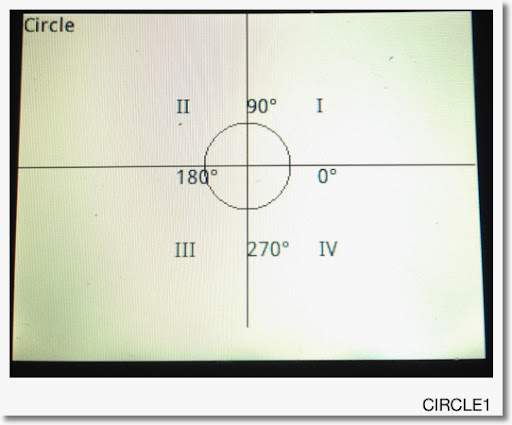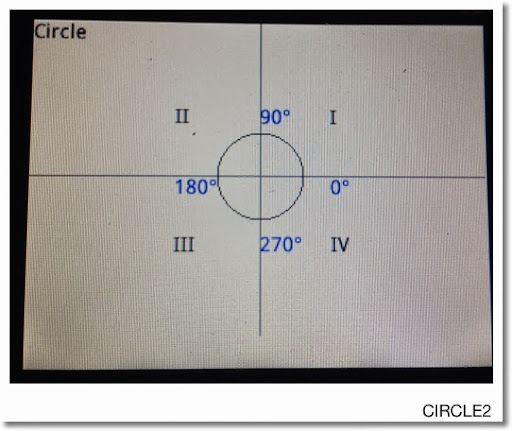## Friday, December 6, 2013

### HP Prime Programming Tutorial #8: ARC, LINE, and Placement of Graphics

Welcome to Part 8 of the HP Prime Tutorial. Continuing in our drawing commands, we are going to feature ARC and LINE.

ARC and ARC_P: Draws a Circle or a Circular Arc

Syntax:

Cartesian:
ARC(x, y, r, angle 1*, angle 2*, color*).

Pixels:
ARC_P(x, y, r, angle 1*, angle 2*, color*)

x: x coordinate

y: y coordinate

r: length of the radius in pixels. This is why I prefer using pixel commands in my programming.

angle 1 and angle 2: If included, these two arguments draw an arc segment. The angle follows the conventional circle notation, starting with 0 (due east) going counter clockwise. Use the current angle mode.

color: color of the arc. To use it, angle 1 and angle 2 must be included.

LINE and LINE_P

Draws a line segment from (x1, y1) to (x2, y2). The color is optional.

Syntax:

Cartesian:
LINE(x1, y1, x2, y2, color*)

Pixels:
LINE_P(x1, y1, x2, y2, color*)

The next to programs CIRCLE1 and CIRCLE2 do the same thing: draw a circle, label quadrants and angels. The reason why I am posting these two is that when you are programming graphics, care must be given. Adjustments to where graphics are placed can be necessary and I hope CIRCLE1 and CIRCLE2 illustrates this.

Medium font is assumed (set in Home Settings).

The angle symbol (°) get be retrieved by pressing Shift + 9 and selecting ° from the grid.

CIRCLE1 (the "rough draft", if you will):

EXPORT CIRCLE1()
BEGIN
RECT();
TEXTOUT_P("Circle", 0, 0);
TEXTOUT_P("II",109,59);
TEXTOUT_P("90°",159,59);
TEXTOUT_P("I",209,59);
TEXTOUT_P("180°",109,109);
TEXTOUT_P("0°",209,109);
TEXTOUT_P("III",109,159);
TEXTOUT_P("270°",159,159);
TEXTOUT_P("IV",209,159);

LINE_P(0,109,318,109,RGB(16,16,16));
LINE_P(159,0,159,239,RGB(16,16,16));

ARC_P(159,109,30);
FREEZE;
END;

The results:This is good, but we can do better. First I am going to move the labels II, 180°, and III 10 pixels to the left, primarily so the 180° label does not touch the circle.

Second I am going to move the labels III, 270°, and IV up 10 pixels to make the bottom line look better and not "so far away" from the circle.

Although it is not necessarily, I am going to make the angle labels blue. I will need to specify the font size code at 0.

Finally, I find that the lines are too dark. I am going to make them a bit more gray.

Here is the second revision. For the purposes of this tutorial, I call this program CIRCLE2. All changes are in bold.

EXPORT CIRCLE1()
BEGIN
RECT();
TEXTOUT_P("Circle", 0, 0);
TEXTOUT_P("II",99,59);
TEXTOUT_P("90°",159,59,0,RGB(0,0,255));
TEXTOUT_P("I",209,59);
TEXTOUT_P("180°",99,109,0,RGB(0,0,255));
TEXTOUT_P("0°",209,109,0,RGB(0,0,255));
TEXTOUT_P("III",99,149);
TEXTOUT_P("270°",159,149,0,RGB(0,0,255));
TEXTOUT_P("IV",209,149);

LINE_P(0,109,318,109,RGB(128,128,128));
LINE_P(159,0,159,239,RGB(128,128,128));

ARC_P(159,109,30);
FREEZE;
END;A better looking picture.

This blog is property of Edward Shore. 2013

Eddie

** Note: Thanks to Dennis for pointing out my typo.  It is corrected now.  3/5/2014

### RCL 40 Book On Sale

RCL 40 Book On Sale The fantastic book, RCL 40: Recollection, Reinvention, & HP Calculators , edited by W.A.C. Mier-Jedrezejowic, Ph. D,...